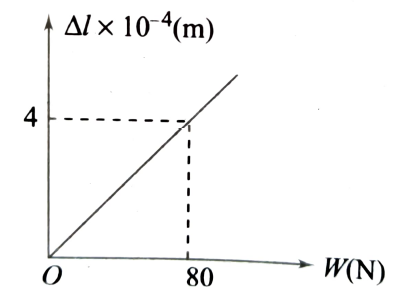Elastic behaviour of solids
Question

# Figure shows a graph of the extension (A) of a wire of length 1 m suspended from the top of a roof at one end and with a load W connected to the other end. If the cross-sectional area of the wire is ${10}^{-6}{m}^{2}$ , the Young's modulus of the material of the wire is $x×{10}^{11}\mathrm{N}/{\mathrm{m}}^{2}$. The value of $x$ isModerate
Solution

## Young's modulus is given by  ……..(i)Now, slope of graph is $\frac{\mathrm{\Delta l}}{\mathrm{W}}=\frac{4×{10}^{-4}}{80}\mathrm{m}/\mathrm{N}$. Using this in i), we get  $\mathrm{Y}=\frac{80}{4×{10}^{-4}}×\frac{1}{{10}^{-6}}=2×{10}^{11}\mathrm{N}/{\mathrm{m}}^{2}$

Get Instant Solutions